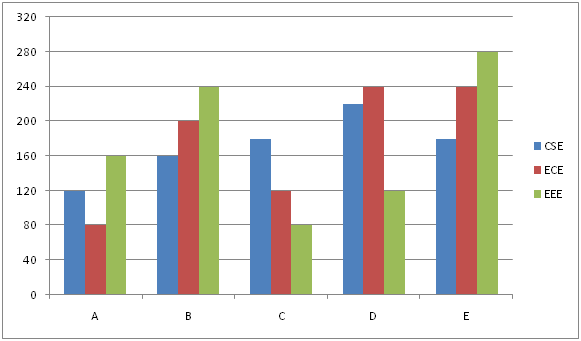# IBPS PO Prelims Quantitative Aptitude Questions 2019 (Day-14)

Dear Aspirants, Our IBPS Guide team is providing new series of Quantitative Aptitude Questions for IBPS PO Prelims 2019 so the aspirants can practice it on a daily basis. These questions are framed by our skilled experts after understanding your needs thoroughly. Aspirants can practice these new series questions daily to familiarize with the exact exam pattern and make your preparation effective.

Check here for IBPS PO Prelims Mock Test 2019

##### Check here for IBPS PO  Mock Test 2019
[WpProQuiz 7143]

Directions (1 – 5): What value should come in the place of question mark in the given questions?

1) 122 + 45% of 120 = 172 – 7 *?

a) 12

b) 14

c) 11

d) 13

e) 15

2) ?2 + √25 * 25 + √81 * 3 = 1521

a) 33

b) 34

c) 37

d) 39

e) 29

3) 60% of 280 + ?% of 320 = 81% of 800

a) 190

b) 180

c) 160

d) 120

e) 150

4) √3025 + ? = 18 * 24 – 30% of 590

a) 180

b) 175

c) 200

d) 220

e) 210

5) (2720 ÷ 34) + 40% of 125 = ?+ √121 * 5 – √225

a) 80

b) 90

c) 70

d) 78

e) 84

Directions (6 – 10): Study the following information carefully and answer the questions given below.

The given bar graph shows the number of students in different colleges in three different departments in 2018.6) Total number of students in A, B and C together in EEE department is what percent of the total number of students in C, D and E in ECE department?

a) 60%

b) 70%

c) 80%

d) 90%

e) 100%

7) What is the difference between the average number of EEE students in all the schools together and the average number of students in B in all the departments together?

a) 13

b) 16

c) 18

d) 22

e) None of these

8) The total number of students in ECE departments in all the colleges together is approximately what percent of the total number of students in C and D together in all the departments together?

a) 88%

b) 90%

c) 78%

d) 86%

e) 92%

9) What is the ratio of the total number of students in CSE department in all the colleges together to total number of students in E in all the departments together?

a) 43: 35

b) 41: 35

c) 45: 37

d) 43: 32

e) None of these

10) What is the difference between the total number of students in A and C in all the departments together?

a) 10

b) 15

c) 20

d) 25

e) 30

Directions (1-5) :

122 + 45% of 120 = 172 – 7 * ?

144 + 54 = 289 – 7 * ?

91 = 7 * ?

? = 13

?2 + √25 * 25 + √81 * 3 = 1521

?2 + 125 + 27 = 1521

?2 = 1369

? = 37

60% of 280 + ?% of 320 = 81% of 800

168 + ? % of 320 = 648

? = 150

√3025 + ? = 18 * 24 – 30% of 590

55 + ? = 432 – 177

? = 200

(2720 ÷ 34) + 40% of 125 = ? + √121 * 5 – √225

80 + 50 = ? + 55 – 15

? = 90

Directions (6-10) :

Required percentage = (160 + 240 + 80)/(120 + 240 + 240) * 100

= 80%

Average number of EEE students = (160 + 240 + 80 + 120 + 280)/5 = 176

Average number of students in B = (160 + 200 + 240)/3 = 200

Difference = 200 – 176 = 24

Total students in ECE department = 80 + 200 + 120 + 240 + 240 = 880

Total students in C and D = 180 + 120 + 80 + 220 + 240 + 120 = 960

Required percentage = 880/960 * 100 = 92%

Required ratio = (120 + 160 + 180 + 220 + 180): (180 + 240 + 280)

= 860: 700

= 43: 35